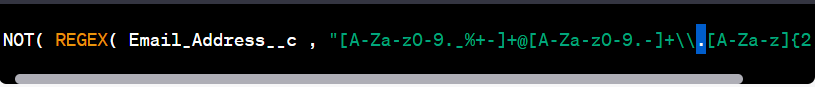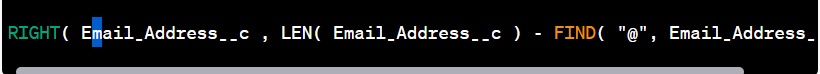# Using Regex in Formula in Salesforce

Salesforce is a popular customer relationship management (CRM) tool that helps businesses manage their customer interactions and data. Within Salesforce, formula fields are a powerful way to manipulate data and display calculated values based on various criteria. Regular expressions (regex) can be used within formula fields to extract and manipulate specific text patterns, allowing for even more advanced calculations and data manipulation. In this blog post, we'll explore how regular expressions are used in formulas in Salesforce, along with a few examples.

What are regular expressions?

Regular expressions (regex) are a set of characters and rules used to define search patterns. They allow for searching, extracting, and manipulating specific patterns of text within a larger body of text. Regular expressions are widely used in programming languages, text editors, and other software to perform powerful text manipulation.

How are regular expressions used in formulas in Salesforce?

Salesforce formula fields allow users to define complex calculations and display calculated values based on various criteria. Regular expressions can be used within formula fields to extract and manipulate specific text patterns. This can be useful for a variety of tasks, such as:

• Extracting specific parts of a text string
• Replacing certain characters or patterns in a text string
• Validating data input by checking if it matches a certain pattern

Let's explore a few examples of how regular expressions can be used in Salesforce formulae.

Example 1: Extracting the first name from a full name

Suppose you have a field called Full Name that contains the full name of a person, and you want to extract just their first name to use in another calculation. You can use the following regular expression in a formula field:Example 1: REGEX( Full_Name__c , "(\\w+)")

This regular expression will match any sequence of word characters (letters, digits, or underscores), and the `REGEX` function will return the first match found. So if the Full Name field contains "John Doe", this formula will return "John".

Example 2: Replacing certain characters in a text string

Suppose you have a field called Phone Number that contains a phone number in the format "(123) 456-7890", and you want to remove the parentheses and hyphen to make it easier to use in a calculation. You can use the following regular expression in a formula field:Example 2: SUBSTITUTE( Phone_Number__c , "[()\\-]", "")

This formula uses the SUBSTITUTE function to replace any occurrences of the characters (, ), or - with an empty string, effectively removing them from the phone number. The regular expression "[()\\-]" matches any occurrence of the characters (, ), or -.

Example 3: Validating data input

Suppose you have a field called Email Address that should only contain valid email addresses, and you want to validate that the input matches the basic structure of an email address. You can use the following regular expression in a validation rule:Example 3: NOT( REGEX( Email_Address__c ,

`"[A-Za-z0-9._%+-]+@[A-Za-z0-9.-]+\\.[A-Za-z]{2,}") )`

This formula uses the REGEX function within a validation rule to check if the input matches the basic structure of an email address. The regular expression

`"[A-Za-z0-9._%+-]+@[A-Za-z0-9.-]+\\.[A-Za-z]{2,}"` matches any string that:

• starts with one or more letters, digits, dots, underscores, percent signs, plus signs, or hyphens, followed by an "@" symbol,
• followed by one or more letters, digits, dots, or hyphens,

Example 4: Extracting the domain name from an email address

Suppose you have a field called Email Address that contains a full email address, and you want to extract just the domain name to use in another calculation. You can use the following regular expression in a formula field:This formula uses the RIGHT function to return the rightmost characters of the email address, starting from the position of the "@" symbol. The LEN function is used to determine the length of the email address, and the FIND function is used to find the position of the "@" symbol.

Example 5: Extracting the numeric part of a string

Suppose you have a field called Order Number that contains a string in the format "ORD-12345", and you want to extract just the numeric part to use in another calculation. You can use the following regular expression in a formula field:Example 5: VALUE( REGEX( Order_Number__c , "\\d+") )

This formula uses the REGEX function to match any sequence of one or more digits, and the VALUE function is used to convert the resulting text string to a numeric value.

Example 6: Removing duplicate words from a string

Suppose you have a field called Description that contains a string with duplicate words, and you want to remove the duplicates to make it easier to read. You can use the following regular expression in a formula field:Example 6: REGEXREPLACE( Description__c , "\\b(\\w+)\\b(?:\\s+\\1\\b)+", "\$1" )

This formula uses the REGEXREPLACE function to replace any occurrences of a word that appears more than once in the string with just one instance of that word. The regular expression \\b(\\w+)\\b(?:\\s+\\1\\b)+ matches any word that appears more than once in the string, and the replacement string \$1 replaces the duplicates with just one instance of the word.

These examples demonstrate just a few of the many ways that regular expressions can be used in Salesforce formulas to extract, manipulate, and validate text data. With a little creativity and practice, you can harness the power of regex to perform even more complex calculations and data manipulation in Salesforce.